• ## FFT

千次阅读 多人点赞 2018-04-11 10:04:56
FFT是离散傅立叶变换的快速算法，可以将一个信号变换到频域。有些信号在时域上是很难看出什么特征的，但是如果变换到频域之后，就很容易看出特征了。这就是很多信号分析采用FFT变换的原因。另外，FFT可以将一个信号...
FFT是离散傅立叶变换的快速算法，可以将一个信号变换到频域。有些信号在时域上是很难看出什么特征的，但是如果变换到频域之后，就很容易看出特征了。这就是很多信号分析采用FFT变换的原因。另外，FFT可以将一个信号的频谱提取出来，这在频谱分析方面也是经常用的。     虽然很多人都知道FFT是什么，可以用来做什么，怎么去做，但是却不知道FFT之后的结果是什意思、如何决定要使用多少点来做FFT。    现在圈圈就根据实际经验来说说FFT结果的具体物理意义。一个模拟信号，经过ADC采样之后，就变成了数字信号。采样定理告诉我们，采样频率要大于信号频率的两倍，这些我就不在此罗嗦了。    采样得到的数字信号，就可以做FFT变换了。N个采样点，经过FFT之后，就可以得到N个点的FFT结果。为了方便进行FFT运算，通常N取2的整数次方。    假设采样频率为Fs，信号频率F，采样点数为N。那么FFT之后结果就是一个为N点的复数。每一个点就对应着一个频率点。这个点的模值，就是该频率值下的幅度特性。具体跟原始信号的幅度有什么关系呢？假设原始信号的峰值为A，那么FFT的结果的每个点（除了第一个点直流分量之外）的模值就是A的N/2倍。而第一个点就是直流分量，它的模值就是直流分量的N倍。而每个点的相位呢，就是在该频率下的信号的相位。第一个点表示直流分量（即0Hz），而最后一个点N的再下一个点（实际上这个点是不存在的，这里是假设的第N+1个点，也可以看做是将第一个点分做两半分，另一半移到最后）则表示采样频率Fs，这中间被N-1个点平均分成N等份，每个点的频率依次增加。例如某点n所表示的频率为：Fn=(n-1)*Fs/N。由上面的公式可以看出，Fn所能分辨到频率为为Fs/N，如果采样频率Fs为1024Hz，采样点数为1024点，则可以分辨到1Hz。1024Hz的采样率采样1024点，刚好是1秒，也就是说，采样1秒时间的信号并做FFT，则结果可以分析到1Hz，如果采样2秒时间的信号并做FFT，则结果可以分析到0.5Hz。如果要提高频率分辨力，则必须增加采样点数，也即采样时间。频率分辨率和采样时间是倒数关系。     假设FFT之后某点n用复数a+bi表示，那么这个复数的模就是An=根号a*a+b*b，相位就是Pn=atan2(b,a)。根据以上的结果，就可以计算出n点（n≠1，且n<=N/2）对应的信号的表达式为：An/(N/2)*cos(2*pi*Fn*t+Pn)，即2*An/N*cos(2*pi*Fn*t+Pn)。对于n=1点的信号，是直流分量，幅度即为A1/N。     由于FFT结果的对称性，通常我们只使用前半部分的结果，即小于采样频率一半的结果。     总结：假设采样频率为Fs，采样点数为N，做FFT之后，某一点n（n从1开始）表示的频率为：Fn=(n-1)*Fs/N；该点的模值除以N/2就是对应该频率下的信号的幅度（对于直流信号是除以N）；该点的相位即是对应该频率下的信号的相位。相位的计算可用函数atan2(b,a)计算。atan2(b,a)是求坐标为(a,b)点的角度值，范围从-pi到pi。要精确到xHz，则需要采样长度为1/x秒的信号，并做FFT。要提高频率分辨率，就需要增加采样点数，这在一些实际的应用中是不现实的，需要在较短的时间内完成分析。解决这个问题的方法有频率细分法，比较简单的方法是采样比较短时间的信号，然后在后面补充一定数量的0，使其长度达到需要的点数，再做FFT，这在一定程度上能够提高频率分辨力。具体的频率细分法可参考相关文献。[附录：本测试数据使用的matlab程序]close all; %先关闭所有图片Adc=2;  %直流分量幅度A1=3;   %频率F1信号的幅度A2=1.5; %频率F2信号的幅度F1=50;  %信号1频率(Hz)F2=75;  %信号2频率(Hz)Fs=256; %采样频率(Hz)P1=-30; %信号1相位(度)P2=90;  %信号相位(度)N=256;  %采样点数t=[0:1/Fs:N/Fs]; %采样时刻%信号S=Adc+A1*cos(2*pi*F1*t+pi*P1/180)+A2*cos(2*pi*F2*t+pi*P2/180);%显示原始信号plot(S);title('原始信号');figure;Y = fft(S,N); %做FFT变换Ayy = (abs(Y)); %取模plot(Ayy(1:N)); %显示原始的FFT模值结果title('FFT 模值');figure;Ayy=Ayy/(N/2);   %换算成实际的幅度Ayy(1)=Ayy(1)/2;F=([1:N]-1)*Fs/N; %换算成实际的频率值plot(F(1:N/2),Ayy(1:N/2));   %显示换算后的FFT模值结果title('幅度-频率曲线图');figure;Pyy=[1:N/2];for i="1:N/2"Pyy(i)=phase(Y(i)); %计算相位Pyy(i)=Pyy(i)*180/pi; %换算为角度end;plot(F(1:N/2),Pyy(1:N/2));   %显示相位图title('相位-频率曲线图');看完这个你就明白谐波分析了。
展开全文• FFT有什么用 快速傅里叶变换 (fast Fourier transform),即利用计算机计算离散傅里叶变换（DFT)的高效、快速计算方法的统称，简称FFT。快速傅里叶变换是1965年由J.W.库利和T.W.图基提出的。采用这种算法能使...
FFT前言

快速傅里叶变换 (fast Fourier transform),即利用计算机计算离散傅里叶变换（DFT)的高效、快速计算方法的统称，简称FFT。快速傅里叶变换是1965年由J.W.库利和T.W.图基提出的。采用这种算法能使计算机计算离散傅里叶变换所需要的乘法次数大为减少，特别是被变换的抽样点数N越多，FFT算法计算量的节省就越显著。
FFT（Fast Fourier Transformation） 是离散傅氏变换（DFT）的快速算法。即为快速傅氏变换。它是根据离散傅氏变换的奇、偶、虚、实等特性，对离散傅立叶变换的算法进行改进获得的。
——百度百科

FFT（Fast Fourier Transformation），中文名快速傅里叶变换，用来加速多项式乘法
朴素高精度乘法时间$O(n^2)$，但$FFT$能$O(n\log_2 n)$的时间解决
$FFT$名字逼格高，也难懂，其他教程写得让人看不太懂，于是自己随便写一下

建议对复数、三角函数相关知识有所耳闻 （不会也无所谓）

下面难懂的点我会从网上盗

多项式的系数表示法和点值表示法

$FFT$其实是一个用$O(n\log_2n)$的时间将一个用系数表示的多项式转换成它的点值表示的算法

多项式的系数表示和点值表示可以互相转换

系数表示法

一个n-1次n项多项式$f(x)$可以表示为$f(x)=\sum^{n-1}_{i=0}a_ix^i$
也可以用每一项的系数来表示$f(x)$，即$f(x)=\{a_0,a_1,a_2,...,a_{n-1} \}$
这就是系数表示法，也就是平时数学课上用的方法

点值表示法

把多项式放到平面直角坐标系里面，看成一个函数

把$n$个不同的$x$代入，会得出$n$个不同的$y$，在坐标系内就是$n$个不同的点

那么这$n$个点唯一确定该多项式，也就是有且仅有一个多项式满足$∀k,f(x_k)=y_k$

理由很简单，把$n$条式子联立起来成为一个有n条方程的n元方程组，每一项的系数都可以解出来

那么$f(x)$还可以用$f(x)=\{(x_0,f(x_0)),(x_1,f(x_1)),(x_2,f(x_2)),...,(x_{n-1},f(x_{n-1}))\}$来表示
这就是点值表示法

高精度乘法下两种多项式表示法的区别
对于两个用系数表示的多项式，我们把它们相乘
设两个多项式分别为$A(x),B(x)$
我们要枚举$A$每一位的系数与$B$每一位的系数相乘
那么系数表示法做多项式乘法时间复杂度$O(n^2)$
但两个用点值表示的多项式相乘，只需要$O(n)$的时间

什么意思呢？

设两个点值多项式分别为$f(x)=\{(x_0,f(x_0)),(x_1,f(x_1)),(x_2,f(x_2)),...,(x_{n-1},f(x_{n-1}))\}$$g(x)=\{(x_0,g(x_0)),(x_1,g(x_1)),(x_2,g(x_2)),...,(x_{n-1},g(x_{n-1}))\}$
设它们的乘积是$h(x)$，那么$h(x)=\{(x_0,f(x_0)·g(x_0)),(x_1,f(x_1)·g(x_1)),...,(x_{n-1},f(x_{n-1})·g(x_{n-1}))\}$
所以这里的时间复杂度只有一个枚举的$O(n)$

突然感觉高精度乘法能$O(n)$暴艹一堆题？

但是朴素的系数表示法转点值表示法的算法还是$O(n^2)$的，逆操作类似

朴素系数转点值的算法叫DFT（离散傅里叶变换），点值转系数叫IDFT（离散傅里叶逆变换）

难道高精度乘法只能$O(n^2)$了吗？

DFT前置知识&技能

复数

毕竟高中有所以不多说

我们把形如a+bi（a,b均为实数）的数称为复数，其中a称为实部，b称为虚部，i称为虚数单位。当虚部等于零时，这个复数可以视为实数；当z的虚部不等于零时，实部等于零时，常称z为纯虚数。复数域是实数域的代数闭包，也即任何复系数多项式在复数域中总有根。 复数是由意大利米兰学者卡当在十六世纪首次引入，经过达朗贝尔、棣莫弗、欧拉、高斯等人的工作，此概念逐渐为数学家所接受。
——百度百科

初中数学老师会告诉你没有$\sqrt{-1}$，但仅限$R$
扩展至复数集$C$，定义$i^2=-1$，一个复数$z$可以表示为$z=a+bi(a,b\in R)$
其中$a$称为实部，$b$称为虚部，$i$称为虚数单位

在复数集中就可以用$i$表示负数的平方根，如$\sqrt{-7}=\sqrt{7}i$

还可以把复数看成复平面直角坐标系上的一个点，比如下面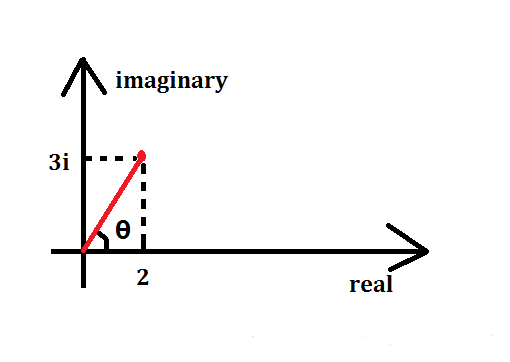$x$轴就是实数集中的坐标轴，$y$轴就是虚数单位$i$轴

这个点$(2,3)$表示的复数就是$2+3i$，或者想象它代表的向量为$(2,3)$
其实我们还可以自己想象 （其实没有这种表达方式） 它可以表示为$(\sqrt{13},\theta)$
一个复数$z$的模定义为它到原点的距离，记为$|z|=\sqrt{a^2+b^2}$
一个复数$z=a+bi$的共轭复数为$a-bi$（虚部取反），记为$\overline{z}=a-bi$

复数的运算

复数不像点或向量，它和实数一样可以进行四则运算
设两个复数分别为$z_1=a+bi,z_2=c+di$，那么
$z_1+z_2=(a+c)+(b+d)i$$z_1z_2=(ac−bd)+(ad+bc)i$
复数相加也满足平行四边形法则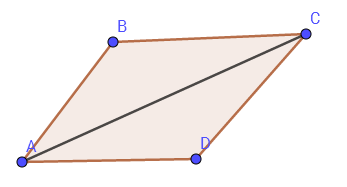这张是从网上盗的

即$AB+AD=AC$
复数相乘还有一个值得注意的小性质
$(a_1,\theta_1)*(a_2,\theta_2)=(a_1a_2,\theta_1+\theta_2)$
即模长相乘，极角相加

DFT（离散傅里叶变换）

一定注意从这里开始所有的$n$都默认为$2$的整数次幂

对于任意系数多项式转点值，当然可以随便取任意$n$个$x$值代入计算
但是暴力计算$x_k^0,x_k^1,...,x_k^{n-1}(k\in[0,n))$当然是$O(n^2)$的时间
其实可以代入一组神奇的$x$，代入以后不用做那么多的次方运算
这些$x$当然不是乱取的，而且取这些$x$值应该就是 傅里叶 的主意了
考虑一下，如果我们代入一些$x$，使每个$x$的若干次方等于$1$，我们就不用做全部的次方运算了
$±1$是可以的，考虑虚数的话$±i$也可以，但只有这四个数远远不够

傅里叶说：这个圆圈上面的点都可以做到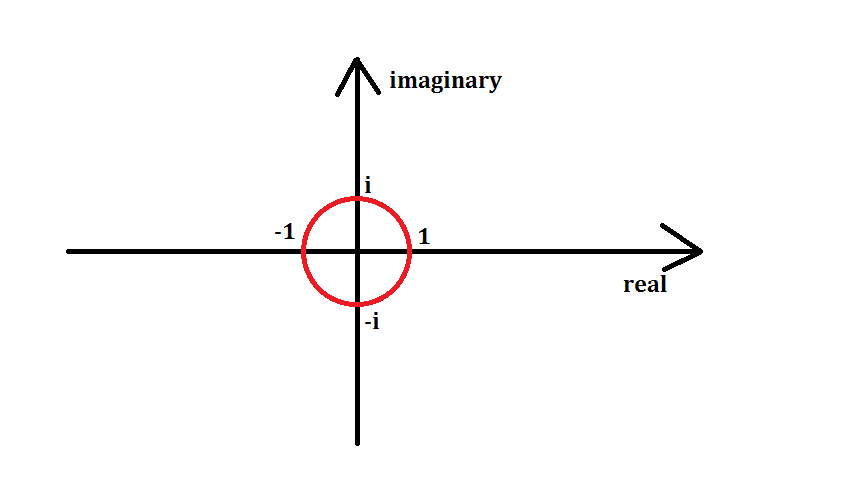以原点为圆心，画一个半径为$1$的单位圆
那么单位圆上所有的点都可以经过若干次次方得到$1$
傅里叶说还要把它给$n$等分了，比如此时$n=8$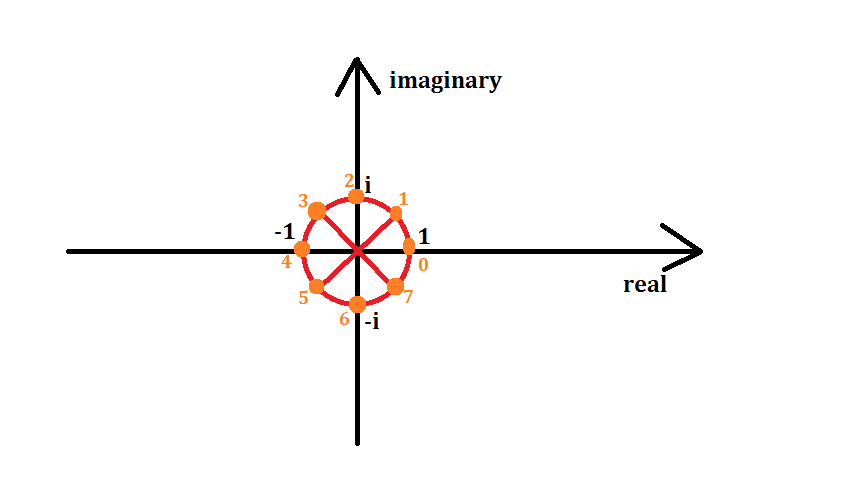橙色点即为$n=8$时要取的点，从$(1,0)$点开始，逆时针从$0$号开始标号，标到$7$号
记编号为$k$的点代表的复数值为$\omega_n^k$，那么由模长相乘，极角相加可知$(\omega_n^1)^k=\omega_n^k$
其中$\omega_n^1$称为$n$次单位根，而且每一个$\omega$都可以求出 （我三角函数不好）
$\omega_n^k=\cos{k\over n}2π+i\sin{k\over n} 2π$
或者说也可以这样解释这条式子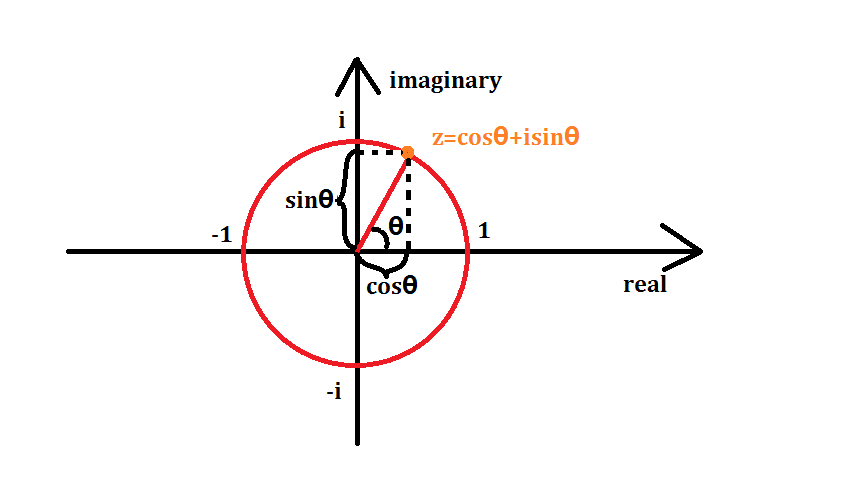注意$sin^2\theta+cos^2\theta=1$什么的，就容易理解了

那么$\omega^0_n,\omega^1_n,...,\omega^{n-1}_n$即为我们要代入的$x_0,x_1,...,x_{n-1}$

单位根的一些性质
推$FFT$的过程中需要用到$\omega$的一些性质

$\omega^k_n=\omega^{2k}_{2n}$

它们表示的点（或向量）表示的复数是相同的

证明

$\omega^k_n=cos{k\over n}2π+isin{k\over n} 2π=cos{2k\over 2n}2π+isin{2k\over 2n} 2π=\omega^{2k}_{2n}$

$\omega^{k+{n \over 2}}_n=-\omega_n^k$

它们表示的点关于原点对称，所表示的复数实部相反，所表示的向量等大反向

证明

$\omega^{n\over 2}_n=cos{{n\over 2}\over n}2\pi+isin{{n\over 2}\over n}2\pi=cos\pi+isin\pi=-1$

（这个东西和$e^{ix}=cosx+isinx$与$e^{i\pi}+1=0$有点关系，我不会就不讲了）

$\omega^0_n=\omega^n_n$

都等于$1$，或$1+0i$

FFT（快速傅里叶变换）
虽然$DFT$搞出来一堆很牛逼的$\omega$作为代入多项式的$x$值
但只是代入这类特殊$x$值法的变换叫做$DFT$而已，还是要代入单位根暴力计算

DFT还是暴力$O(n^2)$…

但$DFT$可以分治来做，于是 FFT（快速傅里叶变换） 就出来了
首先设一个多项式$A(x)$
$A(x)=\sum^{n-1}_{i=0}a_ix^i=a_0+a_1x+a_2x^2+...+a_{n-1}x^{n-1}$
按$A(x)$下标的奇偶性把$A(x)$分成两半，右边再提一个$x$
$A(x)=(a_0+a_2x^2+...+a_{n-2}x^{n-2})+(a_1x+a_3x^3+...+a_{n-1}x^{n-1})$
$=(a_0+a_2x^2+...+a_{n-2}x^{n-2})+x(a_1+a_3x^2+...+a_{n-1}x^{n-2})$
两边好像非常相似，于是再设两个多项式$A_1(x),A_2(x)$，令
$A_1(x)=a_0+a_2x+a_4x^2+...+a_{n-2}x^{{n\over 2}-1}$$A_2(x)=a_1+a_3x+a_5x^2+...+a_{n-1}x^{{n \over 2}-1}$
比较明显得出
$A(x)=A_1(x^2)+xA_2(x^2)$
再设$k<{n\over 2}$，把$\omega^k_n$作为$x$代入$A(x)$（接下来几步变换要多想想单位根的性质）
$A(\omega^k_n)=A_1((\omega^k_n)^2)+\omega^k_nA_2((\omega^k_n)^2)$$=A_1(\omega^{2k}_n)+\omega^k_nA_2(\omega^{2k}_n)=A_1(\omega^k_{n\over2})+\omega^k_nA_2(\omega^k_{n\over 2})$
那么对于$A(\omega^{k+{n\over2}}_n)$的话，代入$\omega^{k+{n \over 2}}_n$
$A(\omega^{k+{n\over 2}}_n)=A_1(\omega^{2k+n}_n)+\omega^{k+{n\over 2}}_nA_2(\omega^{2k+n}_n)$$=A_1(\omega^{2k}_n\omega^n_n)-\omega^k_nA_2(\omega^{2k}_n\omega^n_n)$$=A_1(\omega^{2k}_n)-\omega^k_nA_2(\omega^{2k}_n)=A_1(\omega^k_{n\over2})-\omega^k_nA_2(\omega^k_{n\over2})$

发现了什么？

$A(\omega^k_n)$和$A(\omega^{k+{n\over2}}_n)$两个多项式后面一坨东西只有符号不同
就是说，如果已知$A_1(\omega^k_{n\over 2})$和$A_2(\omega^k_{n\over 2})$的值，我们就可以同时知道$A(\omega^k_n)$和$A(\omega^{k+{n\over2}}_n)$的值
现在我们就可以递归分治来搞$FFT$了
每一次回溯时只扫当前前面一半的序列，即可得出后面一半序列的答案
$n==1$时只有一个常数项，直接$return$
时间复杂度$O(n\log_2n)$

IFFT（快速傅里叶逆变换）
想一下，我们不仅要会$FFT$，还要会IFFT（快速傅里叶逆变换）
我们把两个多项式相乘 （也叫求卷积），做完两遍$FFT$也知道了积的多项式的点值表示
可我们平时用系数表示的多项式，点值表示没有意义

怎么把点值表示的多项式快速转回系数表示法？

$IDFT$暴力$O(n^2)$做？其实也可以用$FFT$用$O(n\log_2n)$的时间搞

你有没有想过为什么傅里叶是把$\omega^k_n$作为$x$代入而不是别的什么数？
原因是有的但是有我也看不懂
由于我是沙雕所以只用记住一个结论

一个多项式在分治的过程中乘上单位根的共轭复数，分治完的每一项除以$n$即为原多项式的每一项系数

意思就是说$FFT$和$IFFT$可以一起搞

朴素版FFT板子
$c++$有自带的复数模板$complex$库
$a.real()$即表示复数$a$的实部
#include<complex>
#define cp complex<double>

void fft(cp *a,int n,int inv)//inv是取共轭复数的符号
{
if (n==1)return;
int mid=n/2;
static cp b[MAXN];
fo(i,0,mid-1)b[i]=a[i*2],b[i+mid]=a[i*2+1];
fo(i,0,n-1)a[i]=b[i];
fft(a,mid,inv),fft(a+mid,mid,inv);//分治
fo(i,0,mid-1)
{
cp x(cos(2*pi*i/n),inv*sin(2*pi*i/n));//inv取决是否取共轭复数
b[i]=a[i]+x*a[i+mid],b[i+mid]=a[i]-x*a[i+mid];
}
fo(i,0,n-1)a[i]=b[i];
}

两个多项式$a,b$相乘再转系数多项式$c$，通常只用打这么一小段
	cp a[MAXN],b[MAXN];
int c[MAXN];
fft(a,n,1),fft(b,n,1);//1系数转点值
fo(i,0,n-1)a[i]*=b[i];
fft(a,n,-1);//-1点值转系数
fo(i,0,n-1)c[i]=(int)(a[i].real()/n+0.5);//注意精度

很明显，FFT只能处理$n$为$2$的整数次幂的多项式
所以在$FFT$前一定要把$n$调到$2$的次幂
这个板子看着好像很优美，但是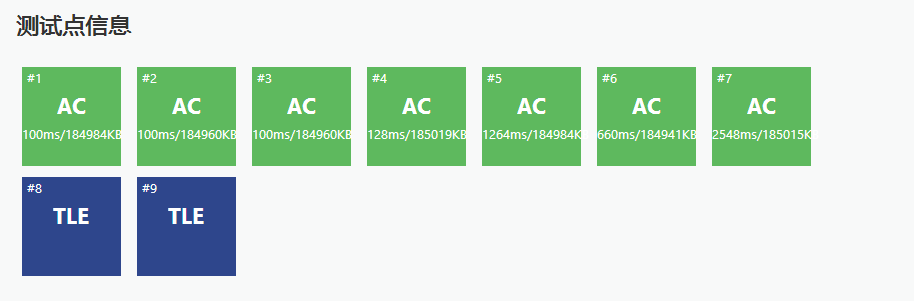递归常数太大，要考虑优化…

FFTの优化——迭代版FFT
这个图也是盗的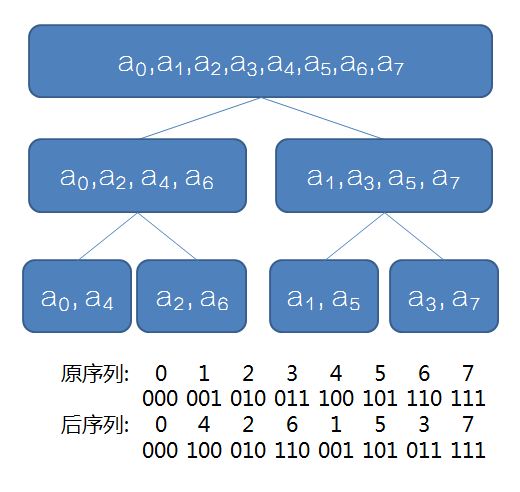这个很容易发现点什么吧？

每个位置分治后的最终位置为其二进制翻转后得到的位置

这样的话我们可以先把原序列变换好，把每个数放在最终的位置上，再一步一步向上合并
一句话就可以$O(n)$预处理第$i$位最终的位置$rev[i]$
fo(i,0,n-1)rev[i]=(rev[i>>1]>>1)|((i&1)<<(bit-1));

至于蝴蝶变换它死了其实是我不会

真·FFT板子
void fft(cp *a,int n,int inv)
{
int bit=0;
while ((1<<bit)<n)bit++;
fo(i,0,n-1)
{
rev[i]=(rev[i>>1]>>1)|((i&1)<<(bit-1));
if (i<rev[i])swap(a[i],a[rev[i]]);//不加这条if会交换两次（就是没交换）
}
for (int mid=1;mid<n;mid*=2)//mid是准备合并序列的长度的二分之一
{
cp temp(cos(pi/mid),inv*sin(pi/mid));//单位根，pi的系数2已经约掉了
for (int i=0;i<n;i+=mid*2)//mid*2是准备合并序列的长度，i是合并到了哪一位
{
cp omega(1,0);
for (int j=0;j<mid;j++,omega*=temp)//只扫左半部分，得到右半部分的答案
{
cp x=a[i+j],y=omega*a[i+j+mid];
a[i+j]=x+y,a[i+j+mid]=x-y;//这个就是蝴蝶变换什么的
}
}
}
}

这个板子好像不是那么好背
至少这个板子已经很优美了

FFT后记
本人版权意识薄弱……

本博客部分知识学习于

https://www.cnblogs.com/RabbitHu/p/FFT.html

https://www.cnblogs.com/zwfymqz/p/8244902.html?mType=Group#_label3

https://blog.csdn.net/ggn_2015/article/details/68922404

$NTT$我来了


展开全文• ## fft

2011-06-10 19:15:00
FFT是离散傅立叶变换的快速算法，可以将一个信号变换到频域。有些信号在时域上是很难看出什么特征的，但是如果变换到频域之后，就很容易看出特征了。这就是很多信号分析采用FFT变换的原因。另外，FFT可以将一个信号...
FFT是离散傅立叶变换的快速算法，可以将一个信号变换到频域。有些信号在时域上是很难看出什么特征的，但是如果变换到频域之后，就很容易看出特征了。这就是很多信号分析采用FFT变换的原因。另外，FFT可以将一个信号的频谱提取出来，这在频谱分析方面也是经常用的。    虽然很多人都知道FFT是什么，可以用来做什么，怎么去做，但是却不知道FFT之后的结果是什意思、如何决定要使用多少点来做FFT。    现在圈圈就根据实际经验来说说FFT结果的具体物理意义。一个模拟信号，经过ADC采样之后，就变成了数字信号。
采样定理告诉我们，采样频率要大于信号频率的两倍，这些我就不在此罗嗦了。采样得到的数字信号，就可以做FFT变换了。N个采样点，经过FFT之后，就可以得到N个点的FFT结果。为了方便进行FFT运算，通常N取2的整数次方。假设采样频率为Fs，信号频率F，采样点数为N。那么FFT之后结果就是一个为N点的复数。每一个点就对应着一个频率点。这个点的模值，就是该频率值下的幅度特性。具体跟原始信号的幅度有什么关系呢？
假设原始信号的峰值为A，那么FFT的结果的每个点（除了第一个点直流分量之外）的模值就是A的N/2倍。而第一个点就是直流分量，它的模值就是直流分量的N倍。而每个点的相位呢，就是在该频率下的信号的相位。第一个点表示直流分量（即0Hz），而最后一个点N的再下一个点（实际上这个点是不存在的，这里是假设的第N+1个点，也可以看做是将第一个点分做两半分，另一半移到最后）则表示采样频率Fs，这中间被N-1个点平均分成N等份，每个点的频率依次增加。例如某点n所表示的频率为：Fn=(n-1)*Fs/N。由上面的公式可以看出，Fn所能分辨到频率为为Fs/N，如果采样频率Fs为1024Hz，采样点数为1024点，则可以分辨到1Hz。1024Hz的采样率采样1024点，刚好是1秒，也就是说，采样1秒时间的信号并做FFT，则结果可以分析到1Hz，如果采样2秒时间的信号并做FFT，则结果可以分析到0.5Hz。如果要提高频率分辨力，则必须增加采样点数，也即采样时间。频率分辨率和采样时间是倒数关系。
假设FFT之后某点n用复数a+bi表示，那么这个复数的模就是An=根号a*a+b*b，相位就是Pn=atan2(b,a)。根据以上的结果，就可以计算出n点（n≠1，且n<=N/2）对应的信号的表达式为：An/(N/2)*cos(2*pi*Fn*t+Pn)，即2*An/N*cos(2*pi*Fn*t+Pn)。对于n=1点的信号，是直流分量，幅度即为A1/N。
由于FFT结果的对称性，通常我们只使用前半部分的结果，即小于采样频率一半的结果。
好了，说了半天，看着公式也晕，下面圈圈以一个实际的信号来做说明。
假设我们有一个信号，它含有2V的直流分量，频率为50Hz、相位为-30度、幅度为3V的交流信号，以及一个频率为75Hz、相位为90度、幅度为1.5V的交流信号。用数学表达式就是如下：S=2+3*cos(2*pi*50*t-pi*30/180)+1.5*cos(2*pi*75*t+pi*90/180)              式中cos参数为弧度，所以-30度和90度要分别换算成弧度。我们以256Hz的采样率对这个信号进行采样，总共采样256点。按照我们上面的分析，Fn=(n-1)*Fs/N，我们可以知道，每两个点之间的间距就是1Hz，第n个点的频率就是n-1。我们的信号有3个频率：0Hz、50Hz、75Hz，应该分别在第1个点、第51个点、第76个点上出现峰值，其它各点应该接近0。实际情况如何呢？我们来看看FFT的结果的模值如图所示。从图中我们可以看到，在第1点、第51点、和第76点附近有比较大的值。我们分别将这三个点附近的数据拿上来细看：
1点： 512+0i
2点： -2.6195E-14 - 1.4162E-13i
3点： -2.8586E-14 - 1.1898E-13i

50点：-6.2076E-13 - 2.1713E-12i
51点：332.55 - 192i
52点：-1.6707E-12 - 1.5241E-12i

75点：-2.2199E-13 -1.0076E-12i
76点：3.4315E-12 + 192i
77点：-3.0263E-14 +7.5609E-13i

很明显，1点、51点、76点的值都比较大，它附近的点值都很小，可以认为是0，即在那些频率点上的信号幅度为0。接着，我们来计算各点的幅度值。分别计算这三个点的模值，
结果如下：
1点： 512
51点：384
76点：192
按照公式，可以计算出直流分量为：512/N=512/256=2；50Hz信号的幅度为：384/(N/2)=384/(256/2)=3；75Hz信号的幅度为192/(N/2)=192/(256/2)=1.5。可见，从频谱分析出来的幅度是正确的。
然后再来计算相位信息。直流信号没有相位可言，不用管它。先计算50Hz信号的相位，atan2(-192, 332.55)=-0.5236,结果是弧度，换算为角度就是180*(-0.5236)/pi=-30.0001。再计算75Hz信号的相位，atan2(192, 3.4315E-12)=1.5708弧度，换算成角度就是180*1.5708/pi=90.0002。可见，相位也是对的。根据FFT结果以及上面的分析计算，我们就可以写出信号的表达式了，它就是我们开始提供的信号。
总结：假设采样频率为Fs，采样点数为N，做FFT之后，某一点n（n从1开始）表示的频率为：Fn=(n-1)*Fs/N；该点的模值除以N/2就是对应该频率下的信号的幅度（对于直流信号是除以N）；该点的相位即是对应该频率下的信号的相位。相位的计算可用函数atan2(b,a)计算。atan2(b,a)是求坐标为(a,b)点的角度值，范围从-pi到pi。要精确到xHz，则需要采样长度为1/x秒的信号，并做FFT。要提高频率分辨率，就需要增加采样点数，
这在一些实际的应用中是不现实的，需要在较短的时间内完成分析。解决这个问题的方法有频率细分法，比较简单的方法是采样比较短时间的信号，然后在后面补充一定数量的0，使其长度达到需要的点数，再做FFT，这在一定程度上能够提高频率分辨力。具体的频率细分法可参考相关文献。

[附录：本测试数据使用的matlab程序]
close all; %先关闭所有图片
Adc=2;  %直流分量幅度
A1=3;   %频率F1信号的幅度
A2=1.5; %频率F2信号的幅度
F1=50;  %信号1频率(Hz)
F2=75;  %信号2频率(Hz)
Fs=256; %采样频率(Hz)
P1=-30; %信号1相位(度)
P2=90;  %信号相位(度)
N=256;  %采样点数
t=[0:1/Fs:N/Fs]; %采样时刻

%信号
S=Adc+A1*cos(2*pi*F1*t+pi*P1/180)+A2*cos(2*pi*F2*t+pi*P2/180);
%显示原始信号
plot(S);
title('原始信号');

figure;
Y = fft(S,N); %做FFT变换
Ayy = (abs(Y)); %取模
plot(Ayy(1:N)); %显示原始的FFT模值结果
title('FFT 模值');

figure;
Ayy=Ayy/(N/2);   %换算成实际的幅度
Ayy(1)=Ayy(1)/2;
F=([1:N]-1)*Fs/N; %换算成实际的频率值
plot(F(1:N/2),Ayy(1:N/2));   %显示换算后的FFT模值结果
title('幅度-频率曲线图');

figure;
Pyy=[1:N/2];
for i="1:N/2"
Pyy(i)=phase(Y(i)); %计算相位
Pyy(i)=Pyy(i)*180/pi; %换算为角度
end;
plot(F(1:N/2),Pyy(1:N/2));   %显示相位图
title('相位-频率曲线图');
展开全文plot matlab 算法 测试
• ## FFT详解

万次阅读 多人点赞 2017-03-31 10:28:34
一直想学FFT，当时由于数学基础太差，导致啥都学不懂。请教了机房里的几位学长大神，结果还是没太明白。因此下定决心写一篇关于“FFT”的文章，一篇起码我能看得懂的“FFT”。
最新更新

2018.6.29 新增了“关于DFT的再次思考” 和 “有关FFT算法实现机理的再讨论”两个小节，希望能对没学明白的同学有所帮助。

2018.6.23 回复了评论区的两个评论，如果同学们觉得我的讲解有错误，或者是有讲得不够清晰的地方，请在评论区评论评论，我会虚心采纳的，谢谢。

2017.12.8 利用LATEX$LATEX$$LATEX$对公式进行重新整理，用以辅助原来的图片公式。但是为了体现出这篇博客的”历史沧桑感”，我保留了原有的所有图片（暂时只完成了一部分的公式转换，公式实在是太多了！）。—— GGN

序言

（来学习的同学们请直接跳过这里就好了…）

非常感谢Leo学长为我耐心的讲解，在此对学长表示诚挚的敬意。停课学习竞赛的那一个月里，学长们不惜耽误自己的时间为我耐心讲解算法，对我的帮助是我永难忘记的。最后衷心祝愿高三学长们在高考中取得优异的成绩！

同时我也要向学长们致歉，感谢学长们曾经对我的包容。

2017.11.19 GGN 补加序言

前言

一直想学FFT，当时由于数学基础太差，导致啥都学不懂。请教了机房里的几位学长大神，结果还是没太明白。因此下定决心写一篇关于“FFT”的文章，一篇起码我能看得懂的“FFT”。不过这好像并不是一件简单的事，因为首先我要学会“FFT”的原理。

建议同学们先自学一下“复数（虚数）”的性质、运算等知识，不然看这篇文章有很大概率看不懂。最后你就会发现，这不是一个“算法”的博客，而是一个数学的博客。

这是当时教我FFT的机房学长的博客：

Leo 的 《FFT与多项式乘法》

（温馨提示：整篇文章都不是很好理解，提醒同学们保持清醒的头脑。）

1.什么是FFT？

FFT，即为快速傅氏变换，是离散傅氏变换的快速算法，它是根据离散傅氏变换的奇、偶、虚、实等特性，对离散傅立叶变换的算法进行改进获得的。它对傅氏变换的理论并没有新的发现，但是对于在计算机系统或者说数字系统中应用离散傅立叶变换，可以说是进了一大步。——360百科

FFT（Fast Fourier Transformation）就是“快速傅里叶变换”的意思，它是一种用来计算DFT（离散傅里叶变换）和IDFT（离散傅里叶反变换）的一种快速算法。这种算法运用了一种高深的数学方式、把原来复杂度为O(n2)$O\left({n}^{2}\right)$$O(n^2)$的朴素多项式乘法转化为了O(nlogn)$O\left(nlogn\right)$$O(nlog n)$的算法。

2.多项式乘法的朴素算法

假设我们现在不会FFT，我们是又是如何计算多项式乘法的呢？现在我们有两个关于x的二次多项式：

f(x)=a1x2+b1x+c1$f\left(x\right)={a}_{1}{x}^{2}+{b}_{1}x+{c}_{1}$f(x)=a_1x^2+b_1x+c_1
g(x)=a2x2+b2x+c2$g\left(x\right)={a}_{2}{x}^{2}+{b}_{2}x+{c}_{2}$g(x)=a_2x^2+b_2x+c_2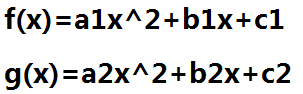我命令K（x）为他们的乘积，则有：

K(x)=f(x)×g(x)=a1a2x4+(a1b2+a2b1)x3+(a1c2+a2c1+b1b2)x2+(b1c2+b2c1)x+c1c2$K\left(x\right)=f\left(x\right)×g\left(x\right)={a}_{1}{a}_{2}{x}^{4}+\left({a}_{1}{b}_{2}+{a}_{2}{b}_{1}\right){x}^{3}+\left({a}_{1}{c}_{2}+{a}_{2}{c}_{1}+{b}_{1}{b}_{2}\right){x}^{2}+\left({b}_{1}{c}_{2}+{b}_{2}{c}_{1}\right)x+{c}_{1}{c}_{2}$K(x)=f(x) \times g(x)=a_1a_2x^4+(a_1b_2+a_2b_1)x^3+(a_1c_2+a_2c_1+b_1b_2)x^2+(b_1c_2+b_2c_1)x+c_1c_2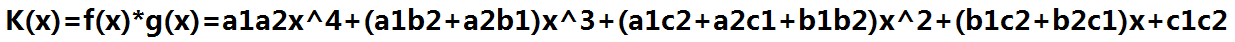如果在程序中，我们用一个数组来储存一个多项式的各个项的系数，我们如何去做这样一个复杂的乘法呢？

#include<iostream>
#include<vector>
#include<cstdlib>
using namespace std;

vector<double>ForceMul(vector<double>A,vector<double>B)//表示A,B两个多项式相乘的结果
{
vector<double>ans;
int aLen=A.size();//A的元素个数
int bLen=B.size();//B的元素个数
int ansLen=aLen+bLen-1;//ans的元素个数=A的元素个数+B的元素个数-1
for(int i=1;i<=ansLen;i++)//初始化ans
ans.push_back(0);
for(int i=0;i<aLen;i++)
for(int j=0;j<bLen;j++)
ans[i+j]+=A[i]*B[j];//A的i次项 与 B的j次项 相乘的结果 累加到ans的[i+j]次位
return ans;//返回ans
}

int main()
{
vector<double>A,B;
cout<<"input A:";
for(int i=0;i<3;i++)//从0次项开始输入A的各项系数
{
int x;
cin>>x;
A.push_back(x);
}
cout<<"input B:";
for(int i=0;i<3;i++)//从0次项开始输入B的各项系数
{
int x;
cin>>x;
B.push_back(x);
}
vector<double>C=ForceMul(A,B);//C=A与B暴力相乘
cout<<"output C:";
for(int i=0;i<5;i++)//从0次项开始输出C的各项系数
cout<<C[i]<<" ";
cout<<endl;
system("pause");
return 0;
}


这就是朴素算法,它的复杂度为O（lenA*lenB）。如果lenA=lenB=10^5，程序时间就会爆掉，那么我们如何进行优化呢？

3.系数表示法与点值表示法

系数表表示法，就是用一个多项式的各个项的系数表示这个多项式，也就是我们平时所用的表示法。例如，我们可以这样表示：

f(x)=a0+a1x+a2x2+..+anxn⇔f(x)={a0,a1,a2,..,an}$f\left(x\right)={a}_{0}+{a}_{1}x+{a}_{2}{x}^{2}+..+{a}_{n}{x}^{n}⇔f\left(x\right)=\left\{{a}_{0},{a}_{1},{a}_{2},..,{a}_{n}\right\}$f(x)=a_0+a_1x+a_2x^2+..+a_nx^n \Leftrightarrow f(x)=\{a_0,a_1,a_2,..,a_n\}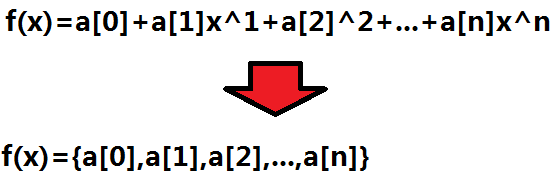点值表示法，就是把这个多项式理解成一个函数，用这个函数上的若干个点的坐标来描述这个多项式。

（两点确定一条直线，三点确定一条抛物线…同理n+1个点确定一个n次函数，其原理来自于“高斯消元”，下文会有介绍。）

因此表示成这样：（注意：x->x[n]是n+1个点）

f(x)=a0+a1x+a2x2+..+anxn⇔f(x)={(x0,y0),(x1,y1),(x2,y2),..,(xn,yn)}$f\left(x\right)={a}_{0}+{a}_{1}x+{a}_{2}{x}^{2}+..+{a}_{n}{x}^{n}⇔f\left(x\right)=\left\{\left({x}_{0},{y}_{0}\right),\left({x}_{1},{y}_{1}\right),\left({x}_{2},{y}_{2}\right),..,\left({x}_{n},{y}_{n}\right)\right\}$f(x)=a_0+a_1x+a_2x^2+..+a_nx^n \Leftrightarrow f(x)=\{(x_0,y_0),(x_1,y_1),(x_2,y_2),..,(x_n,y_n)\}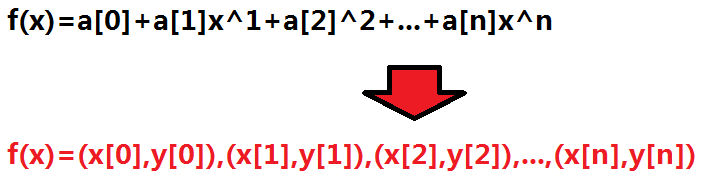为什么n+1个确定的点能确定一个唯一的多项式呢？你可以尝试着把这n+1个点的值分别代入多项式中：

f(x0)=y0=a0+a1x0+a2x20+..+anxn0$f\left({x}_{0}\right)={y}_{0}={a}_{0}+{a}_{1}{x}_{0}+{a}_{2}{x}_{0}^{2}+..+{a}_{n}{x}_{0}^{n}$f(x_0)=y_0=a_0+a_1x_0+a_2x_0^2+..+a_nx_0^n

f(x1)=y1=a0+a1x1+a2x21+..+anxn1$f\left({x}_{1}\right)={y}_{1}={a}_{0}+{a}_{1}{x}_{1}+{a}_{2}{x}_{1}^{2}+..+{a}_{n}{x}_{1}^{n}$f(x_1)=y_1=a_0+a_1x_1+a_2x_1^2+..+a_nx_1^n

f(x2)=y2=a0+a1x2+a2x22+..+anxn2$f\left({x}_{2}\right)={y}_{2}={a}_{0}+{a}_{1}{x}_{2}+{a}_{2}{x}_{2}^{2}+..+{a}_{n}{x}_{2}^{n}$f(x_2)=y_2=a_0+a_1x_2+a_2x_2^2+..+a_nx_2^n

...$...$...

f(xn)=yn=a0+a1xn+a2x2n+..+anxnn$f\left({x}_{n}\right)={y}_{n}={a}_{0}+{a}_{1}{x}_{n}+{a}_{2}{x}_{n}^{2}+..+{a}_{n}{x}_{n}^{n}$f(x_n)=y_n=a_0+a_1x_n+a_2x_n^2+..+a_nx_n^n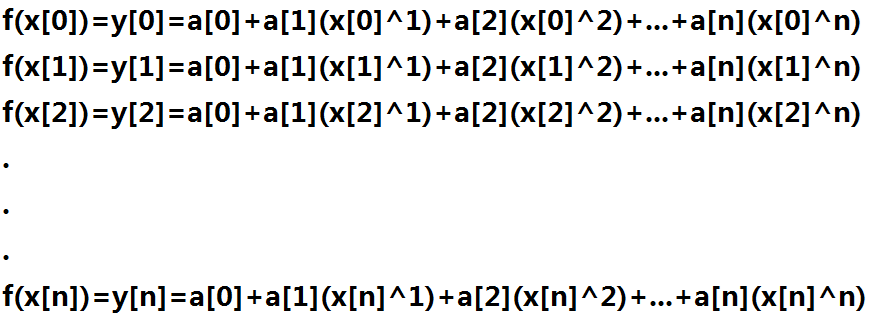你会得到n+1个方程，其中x[0~n]和y[0~n]是已知的，a[0~n]是未知的。n+1的未知数，n+1个方程所组成的方程组为“n+1元一次方程”，因为它是“一次方程”，所以（一般情况下，不考虑无解和无数解）可以通过“高斯消元”解得所有未知数唯一确定的值。也就是说，用点知表示法可以确定出 唯一确定的 系数表示法中的 每一位的 系数。

这种把一个多项式转化成“离散的点”表示的方法就叫做“DFT”（离散傅里叶变换）。

把“离散的点”还原回多项式的方法就叫做“IDFT”（离散傅里叶反变换）。

（请同学们重视上面的这两句话，因为这是我能想到的最好理解的解释方法了。）

有兴趣的可以看一下360百科给出的DFT定义：

离散傅里叶变换（Discrete Fourier Transform，缩写为DFT），是傅里叶变换在时域和频域上都呈离散的形式，将信号的时域采样变换为其DTFT的频域采样。在形式上，变换两端（时域和频域上）的序列是有限长的，而实际上这两组序列都应当被认为是离散周期信号的主值序列。即使对有限长的离散信号作DFT，也应当将其看作其周期延拓的变换。在实际应用中通常采用快速傅里叶变换计算DFT。——360百科

4.“复数”的引入

初中我们可能学过一些定理：比如−1−−−√$\sqrt{-1}$$\sqrt{-1}$不存在。但是，当时我们的“数域”仅仅止步于“实数”，而现在我们要介绍一个新的数域——“虚数”。我们令：i=−1−−−√$i=\sqrt{-1}$$i=\sqrt{-1}$表示这个“虚数单位”，“i$i$$i$”对于虚数的意义就相当于是“数字1”对于实数的意义。

这是百科对“虚数”的定义：

虚数可以指不实的数字或并非表明具体数量的数字。——360百科

在数学中，虚数就是形如a+b*i的数，其中a,b是实数，且b≠0,i² = - 1。虚数这个名词是17世纪著名数学家笛卡尔创立，因为当时的观念认为这是真实不存在的数字。后来发现虚数a+b*i的实部a可对应平面上的横轴虚部b与对应平面上的纵轴，这样虚数a+b*i可与平面内的点(a,b)对应。——360百科

然后是百科对“复数”的定义：

复数x被定义为二元有序实数对(a,b) ，记为z=a+bi,这里a和b是实数，i是虚数单位。在复数a+bi中，a=Re(z)称为实部，b=Im(z)称为虚部。当虚部等于零时，这个复数可以视为实数;当z的虚部不等于零时，实部等于零时，常称z为纯虚数。复数域是实数域的代数闭包，也即任何复系数多项式在复数域中总有根。 复数是由意大利米兰学者卡当在十六世纪首次引入，经过达朗贝尔、棣莫弗、欧拉、高斯等人的工作，此概念逐渐为数学家所接受。

正如上文所说，一个复数可以看成是“复平面”上的一个点。复平面就是以实数部为x轴，以虚部为y轴所组成的类似“直角坐标系”的一个平面坐标体系。同样，我们也可以用“极坐标”来表示一个平面中的点。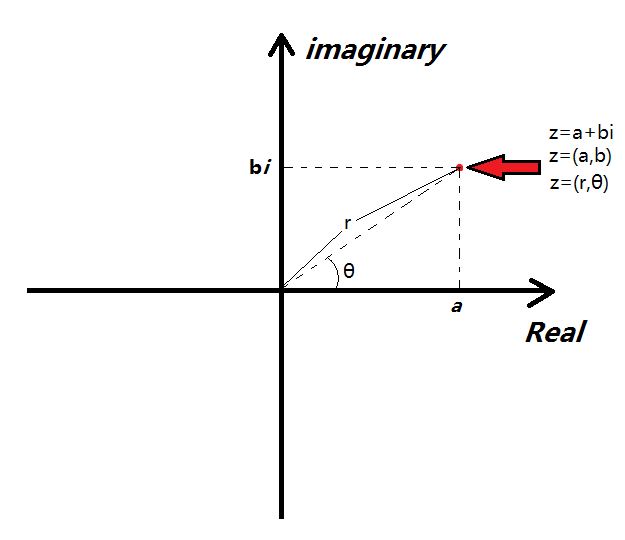上图就是在这个复平面上的一个点的三种表示方法。

然后，思考一个简单的问题：两个复数的乘法有没有某种特定的几何意义？（只是一个数学性质，在此不进一步深究，可用三角函数证明。）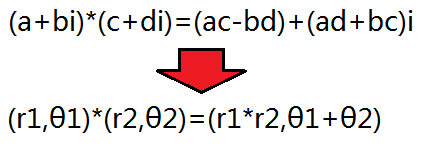两复数相乘，“长度相乘，极角相加”。不难想象如果两个复数它们到“坐标原点”的距离都是1，那么它们的乘积到坐标原点的距离还是一，只不过是绕着原点进行了旋转。

5.单位复根

现在，回到我们刚才讲到的“点值表示法”的问题，考虑这样一个问题，如果我有两个用点值表示的多项式，如何表示它们两个多项式的乘积呢？（假设这两个多项式选取的所有点的x值恰好相同。）

f(x)={(x0,f(x0)),(x1,f(x1)),(x2,f(x2)),..,(xn,f(xn))}$f\left(x\right)=\left\{\left({x}_{0},f\left({x}_{0}\right)\right),\left({x}_{1},f\left({x}_{1}\right)\right),\left({x}_{2},f\left({x}_{2}\right)\right),..,\left({x}_{n},f\left({x}_{n}\right)\right)\right\}$f(x)=\{(x_0,f(x_0)),(x_1,f(x_1)),(x_2,f(x_2)),..,(x_n,f(x_n))\}

g(x)={(x0,g(x0)),(x1,g(x1)),(x2,g(x2)),..,(xn,g(xn))}$g\left(x\right)=\left\{\left({x}_{0},g\left({x}_{0}\right)\right),\left({x}_{1},g\left({x}_{1}\right)\right),\left({x}_{2},g\left({x}_{2}\right)\right),..,\left({x}_{n},g\left({x}_{n}\right)\right)\right\}$g(x)=\{(x_0,g(x_0)),(x_1,g(x_1)),(x_2,g(x_2)),..,(x_n,g(x_n))\}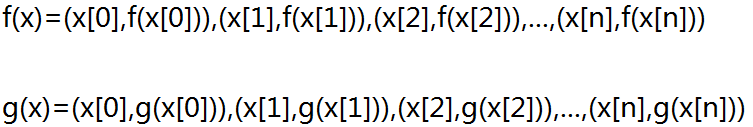如果F(x)=f(x)×g(x)$F\left(x\right)=f\left(x\right)×g\left(x\right)$$F(x)=f(x) \times g(x)$，那么就有F(x0)=f(x0)∗g(x0)$F\left({x}_{0}\right)=f\left({x}_{0}\right)\ast g\left({x}_{0}\right)$$F(x_0)=f(x_0)*g(x_0)$（x0${x}_{0}$$x_0$为任意数）。也就是说，如果我把两个函数的点值表示法中的x$x$$x$值相同的点的y值乘在一起就是它们的乘积（新函数）的点值表示。（这是一个O(n)$O\left(n\right)$$O(n)$的操作。）

f(x)×g(x)={(x0,f(x0)g(x0)),(x1,f(x1)g(x1)),(x0,f(x2)g(x2)),..,(xn,f(xn)g(xn))}$f\left(x\right)×g\left(x\right)=\left\{\left({x}_{0},f\left({x}_{0}\right)g\left({x}_{0}\right)\right),\left({x}_{1},f\left({x}_{1}\right)g\left({x}_{1}\right)\right),\left({x}_{0},f\left({x}_{2}\right)g\left({x}_{2}\right)\right),..,\left({x}_{n},f\left({x}_{n}\right)g\left({x}_{n}\right)\right)\right\}$f(x)\times g(x)=\{(x_0,f(x_0)g(x_0)),(x_1,f(x_1)g(x_1)),(x_0,f(x_2)g(x_2)),..,(x_n,f(x_n)g(x_n))\}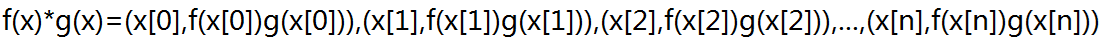但是我们要的是系数表达式，而不是点制表达式，如果用高斯消元暴力地去解一个“n+1元方程组”恐怕就要把时间复杂度拉回O(n2)$O\left({n}^{2}\right)$$O(n^2)$（甚至更高）。为什么呢？因为当我们计算x0，x20，...，xn0${x}_{0}，{x}_{0}^{2}，...，{x}_{0}^{n}$$x_0，x_0^2，...，x_0^n$时会浪费大量的时间。这个数学运算看似是没有办法加速的，而实际上我们可以找到一种神奇的“x值”，带进去之后不用反复地去做无用n次方操作。我能想到的第一个数就是“1”，因为1的几次方都是1。然后我能想到的数就是“-1”，因为“-1”的平方是1。把这样的数带进去就可以减少我们的运算次数。

但是问题又来了，我们只有“-1”和“1”两个数，但是我们要至少带进去n+1个不同的数才能进行系数表示。考虑一个问题：也许“虚数”可能会帮上我们大忙。我们需要的是满足“ωk=1${\omega }^{k}=1$$\omega ^k=1$”的数（k为整数），然后我们就会发现“i”好像也满足这个条件：i*i=-1，（i*i）*（i*i）=1=i^4，当然“-i”也有这个性质。然而仅仅4个数还是不能满足我们的需求。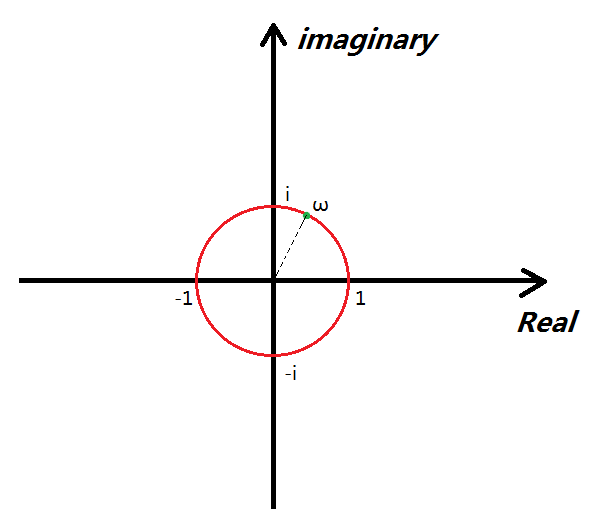看上图中的红圈，红圈上的每一个点距原点的距离都是1个单位长度，所以说如果说对这些点做k次方运算，它们始终不会脱离这个红圈。因为它们在相乘的时候r始终=1，只是θ的大小在发生改变。而这些点中有无数个点经过若干次方之后可以回到“1”。因此，我们可以把这样的一组神奇的x带入函数求值。

像这种能够通过k次方运算回到“1”的数，我们叫它“复根”用“ω”表示。如果ωk=1${\omega }^{k}=1$$\omega ^k=1$，那么我们称“ω$\omega$$\omega$为1的k次复根”计做“ωnk${\omega }_{k}^{n}$$\omega_k^n$”:其中“n”就是一个序号数，我们把所有的“负根”按照极角大小逆时针排序从零开始编号。以“四次负根”“ωn4${\omega }_{4}^{n}$$\omega_4^n$”为例：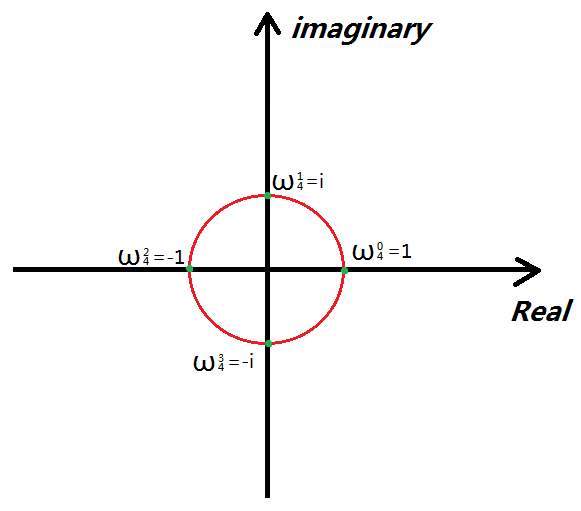你会发现：其实k次负根就相当于是给图中的圆周平均分成k个弧，弧与弧之间的端点就是“复根”，另外ω24=−1=i2=(ω14)2,ω34=−i=i3=(ω14)3${\omega }_{4}^{2}=-1={i}^{2}=\left({\omega }_{4}^{1}{\right)}^{2},{\omega }_{4}^{3}=-i={i}^{3}=\left({\omega }_{4}^{1}{\right)}^{3}$$\omega_4^2=-1=i^2=(\omega_4^1)^2,\omega_4^3=-i=i^3=(\omega_4^1)^3$，ω04${\omega }_{4}^{0}$$\omega_4^0$是这个圆与“Real”轴正半轴的交点，所以无论k取多少，ω04${\omega }_{4}^{0}$$\omega_4^0$始终=1。我们只需要知道ω14${\omega }_{4}^{1}$$\omega_4^1$，就能求出ωnk${\omega }_{k}^{n}$$\omega_k^n$，所以我们称“ω1k${\omega }_{k}^{1}$$\omega_k^1$”为“单位复根”。

其实，我们用“ωk${\omega }_{k}$$\omega_k$”表示单位复根，ω1k${\omega }_{k}^{1}$$\omega_k^1$表示的是“单位复根”的“1次方”也就是它本身,其他的就叫做k次单位复根的n次方。6.FFT的主要流程之DFT

说了这么多了，终于说到FFT了。FFT运用到了一种分治的思想，分治地去求当x=ω(k)[n]时整个多项式的值（永远都不要忘了每一个步骤的目的是什么）。你可以把一个多项式分成奇数次数项，和偶数次数项两部分，然后再用分治的思想去处理它的“奇数次项”和“偶数次项”。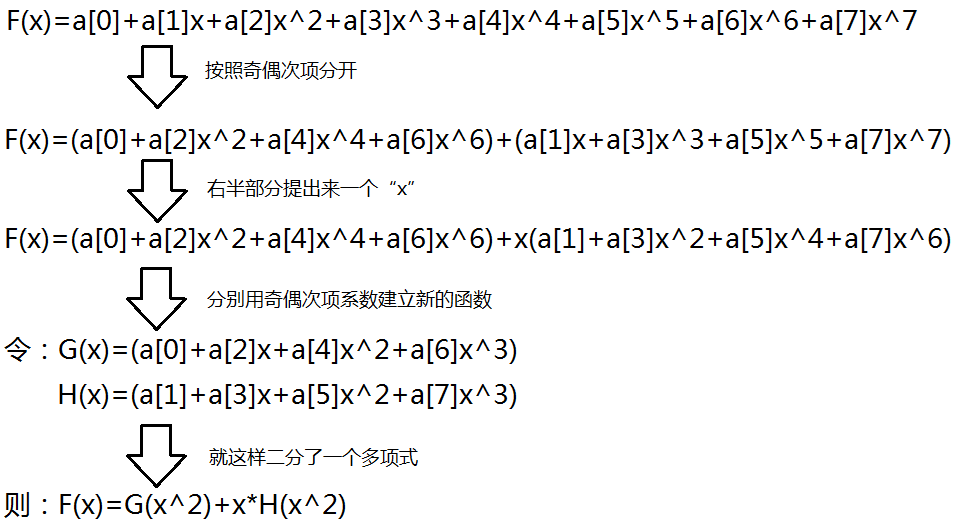我们用DFT(F(x))[k]表示当x=ω^k时F(x)的值，所以有：DFT(F(x))[k]=DFT(G(x^2))[k]+ω^k*DFT(H(x^2))，也就是：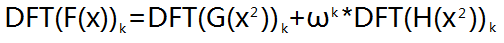（把当前单位复根的平方分别以DFT的方式带入G函数和H函数求值。）

但是这个二分最大的局限就是只能处理长度为2的整数次幂的多项式，因为如果长度不为的整数次幂，二分到最后就会出现左半部分右半部分长度不一致的情况（导致程序取不到系数而爆炸），所以我们在做第一次DFT之前一定要把这个多项式补成长度为2^n（n为整数）的多项式（补上去的高次项系数为“0”），长度为2^n的多项式的最高次项为2^n-1次项。当我们向这个式子中“带入数值”的时候，一定要保证我带入的每个数都是不一样的，所以要带入1的2^n的单位复根的各个幂（因为1的2^n复根恰好有2^n个）。

【2018.6.30】 我觉得上面这一段内容讲得还不够清晰，如果同学们没明白这段话的意思，可以先跳到后面“关于DFT的再次思考”，看看那里的讲解。看完以后再回到这里，继续阅读。

但是这个算法还需要从“分治”的角度继续优化。我们每一次都会把整个多项式的奇数次项和偶数次项系数分开，一只分到只剩下一个系数。但是，这个递归的过程需要更多的内存。因此，我们可以先“模仿递归”把这些系数在原数组中“拆分”，然后再“倍增”地去合并这些算出来的值。然而我们又要如何去拆分这些数呢？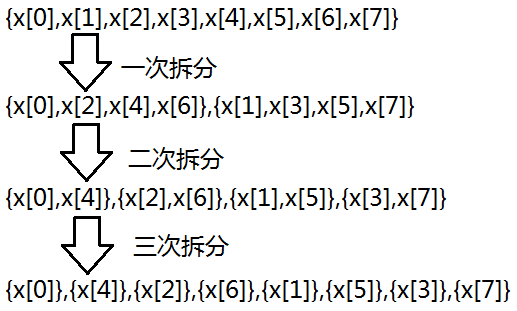貌似并没有什么规律可循，但实际上你可要看仔细了，把这些下标都转化成二进制看看吧：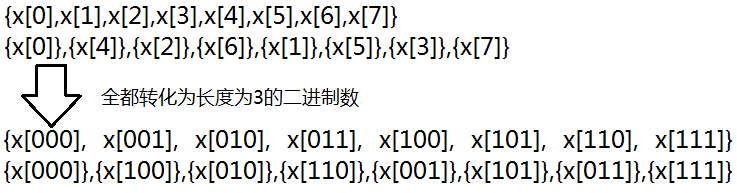是不是发现了一些神奇的特点：“拆分”之后的序列的下标恰好为长度为3位的二进制数的翻转。也就是说我们对原来的每个数的下标进行长度为三的二进制翻转就是新的下标。而为什么是长度为3呢？因为8=2^3。为了证明这一点，我们可以再举一个简单例子（它还是成立的）：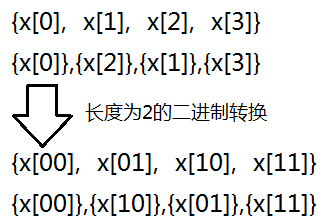（一个数学性质，在此不再证明。我感觉这个原理有点像是“基数排序”，感兴趣的同学可以去看看。）

关于二进制翻转是如何实现的，本文中并没有介绍，强烈建议看一下这篇文章：

学习链接：补充——FFT中的二进制翻转问题

7.FFT的主要流程之IDFT

我们先整理一下思路，IDFT是做什么的？IDFT（傅里叶反变换）就是把一个用“点值表示法”表示的多项式，转化成一个用“系数表示法”表示的多项式，但是这似乎并不是很容易。然而其实我们刚刚恰好做了一些非常机智的事情——把“单位复根”的若干次方带入了原多项式。我们可以表示一下这些多项式（这里使用一个矩阵表示，不会的建议自学）。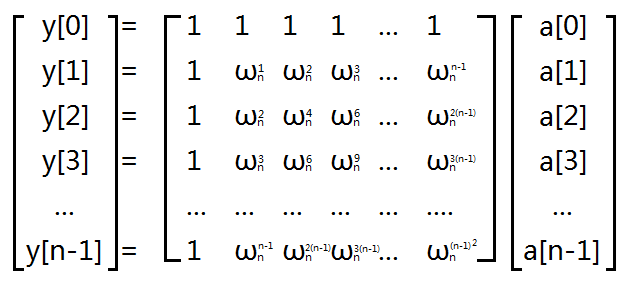如果我们想把这个表达式还原成只含有“a系数”的矩阵，那么就要在中间那个“巨大的矩阵”身上乘上一个它的“反矩阵”（反对称矩阵）就可以了。这个矩阵的中有一种非常特殊的性质，对该矩阵的每一项取倒数，再除以n就可以得到该矩阵的反矩阵。而如何改变我们的操作才能使计算的结果文原来的倒数呢？这就要看我们求“单位复根的过程了”：根据“欧拉函数”e^iπ=-1，我么可以得到e^2πi=1。如果我要找到一个数，它的k次方=1，那么这个数ω[k]=e^(2πi/k)（因为(e^(2πi/k))^k=e^(2πi)=1）。而如果我要使这个数值变成“1/ω[k]”也就是“(ω[k])^-1”，我们可以尝试着把π取成-3.14159…，这样我们的计算结果就会变成原来的倒数，而其它的操作过程与DFT是完全相同的（这真是极好的）。我们可以定义一个函数，向里面掺一个参数“1”或者是“-1”，然后把它乘到“π”的身上。传入“1”就是DFT，传入“-1”就是IDFT，十分的智能。

机房学长的代码写得实在是太好了Orz，忍不住引用了一下：

下面的代码来自 Leo的 《FFT与多项式乘法》

欢迎同学们一同Orz ↑

这是整个代码的一个局部（出于礼貌，保留了原有的代码格式，但是加了一些注释）：

int rev[maxl];
void get_rev(int bit)//bit表示二进制位数,计算一个数在二进制翻转之后形成的新数
{
for(int i=0;i<(1<<bit);i++)
rev[i]=(rev[i>>1]>>1)|((i&1)<<(bit-1));
}
void fft(cd *a,int n,int dft)//n表示我的多项式位数
{
for(int i=0;i<n;i++) if(i<rev[i]) swap(a[i],a[rev[i]]);
//中间的那个if保证了每个数做多只被交换了1次
//如果不写那么会有一些数被交换两次,导致最终的位置没有变
for(int step=1;step<n;step<<=1)//模拟一个合并的过程
{
cd wn=exp(cd(0,dft*PI/step));//计算当前单位复根
for(int j=0;j<n;j+=step<<1)
{
cd wnk(1,0);//计算当前单位复根
for(int k=j;k<j+step;k++)
{//蝴蝶操作
cd x=a[k];
cd y=wnk*a[k+step];
a[k]=x+y;//这就是上文中F(x)=G(x)+ωH(x)的体现
a[k+step]=x-y;
//后半个“step”中的ω一定和“前半个”中的成相反数
//“红圈”上的点转一整圈“转回来”，转半圈正好转成相反数
wnk*=wn;
}
}
}
if(dft==-1) for(int i=0;i<n;i++) a[i]/=n;
//考虑到如果是IDFT操作,整个矩阵中的内容还要乘上1/n
}

关于DFT的再次思考

2018.6.29 我最近有思考了一些关于DFT原理的问题，觉得之前讲得有些草率，决定再写一个表述更清晰一些的版本。每个人都有适合的自己讲课风格，如果没看懂上面的讲解，不妨试试看看这里。

说到DFT（离散傅里叶变换）这个环节，就必须先说这个环节的目的。做音频处理的人用这个过程来实现值域与频域的转换，而我们OIer主要是为了把一个用系数表示法表示的函数转化为一个用点值表示法表示的函数。

我在前文中提到了一类神奇的数——单位复根，并且了解了关于它的一些性质：
1.我们通常用 ωn=e2πin${\omega }_{n}={e}^{\frac{2\pi i}{n}}$$\omega_n = e^{\frac{2\pi i}{n}}$表示n次单位复根（的一次方）

2.n次单位复根的值随指数变化而循环，有 ωn+kn=ωkn${\omega }_{n}^{n+k}={\omega }_{n}^{k}$$\omega_n^{n+k}=\omega_n^k$，（也就是说k为整数时，ωkn${\omega }_{n}^{k}$$\omega_n^k$恰有n种不同的取值，分别为ω0n,ω1n,ω2n,...,ωn−1n${\omega }_{n}^{0},{\omega }_{n}^{1},{\omega }_{n}^{2},...,{\omega }_{n}^{n-1}$$\omega_n^0,\omega_n^1,\omega_n^2,...,\omega_n^{n-1}$，k等于其余整数值时，都可以通过几次+n或者-n 平移 到这个区间里）

3.折半引理： ωkn=e2πin⋅k=e2πin2⋅k2=ωk2n2${\omega }_{n}^{k}={e}^{\frac{2\pi i}{n}\cdot k}={e}^{\frac{2\pi i}{\frac{n}{2}}\cdot \frac{k}{2}}={\omega }_{\frac{n}{2}}^{\frac{k}{2}}$$\omega_n^k = e^{\frac{2\pi i}{n} \cdot k} = e^{\frac{2\pi i}{\frac{n}{2}} \cdot \frac{k}{2}} = \omega_{\frac{n}{2}}^{\frac{k}{2}}$，这个性质一会儿会用到，一定要理解透彻。

一个n-1次多项式可以用n个系数去描述，也可以用函数上的n个点去描述，n+1个点当然也可以，但是多余。根据上述2号结论，我们知道n次复根恰好有n种不同的取值，那我们不妨就把这n个不同的值带入多项式。

这样实际上，问题就转化成——把一个长度为n的向量A，变换成另一个长度为n的向量B。（我们记A与B的下标从0开始，到n-1结束，共n个位置。）

其中：
A 为系数向量，Ak${A}_{k}$$A_k$ 中储存原多项式中xk${x}^{k}$$x^k$项前的系数。
B 为点值向量，Bk${B}_{k}$$B_k$ 中储存 x=ωkn$x={\omega }_{n}^{k}$$x = \omega_n^k$ 时原多项式的值。

为了描述方便，我们称 B向量 为多项式 A0+A1x+A2x2+...+An−1xn−1${A}_{0}+{A}_{1}x+{A}_{2}{x}^{2}+...+{A}_{n-1}{x}^{n-1}$$A_0 + A_1x + A_2 x^2 +...+A_{n-1}x^{n-1}$的变换结果。

根据这个定义，我们可以表示出B向量某一个位置k上的值Bk${B}_{k}$$B_k$：

Bk=A0+A1⋅(ωkn)+A2⋅(ωkn)2+A3⋅(ωkn)3+...+An−1⋅(ωkn)n−1${B}_{k}={A}_{0}+{A}_{1}\cdot \left({\omega }_{n}^{k}\right)+{A}_{2}\cdot \left({\omega }_{n}^{k}{\right)}^{2}+{A}_{3}\cdot \left({\omega }_{n}^{k}{\right)}^{3}+...+{A}_{n-1}\cdot \left({\omega }_{n}^{k}{\right)}^{n-1}$$B_k = A_0 + A_1 \cdot (\omega_n^k) + A_2 \cdot(\omega_n^k)^2 + A_3 \cdot(\omega_n^k)^3+...+A_{n-1}\cdot(\omega_n^k)^{n-1}$

我们不妨假设n为偶数（因为多向式可以在最后补上一些系数为0的高次项，所以这一假设对运算结果没有影响），我们把这个多项式的奇数次项和偶数次项分开，看看会不会得到一些惊人的结论。

推导（因为n为偶数，所以n-2为偶数、n-1为奇数）：

Bk=(A0+A2⋅(ωkn)2+A4⋅(ωkn)4...+An−2⋅(ωkn)n−2)+(A1⋅(ωkn)+A3⋅(ωkn)3+A5⋅(ωkn)5+...+An−1⋅(ωkn)n−1)${B}_{k}=\left({A}_{0}+{A}_{2}\cdot \left({\omega }_{n}^{k}{\right)}^{2}+{A}_{4}\cdot \left({\omega }_{n}^{k}{\right)}^{4}...+{A}_{n-2}\cdot \left({\omega }_{n}^{k}{\right)}^{n-2}\right)+\left({A}_{1}\cdot \left({\omega }_{n}^{k}\right)+{A}_{3}\cdot \left({\omega }_{n}^{k}{\right)}^{3}+{A}_{5}\cdot \left({\omega }_{n}^{k}{\right)}^{5}+...+{A}_{n-1}\cdot \left({\omega }_{n}^{k}{\right)}^{n-1}\right)$$B_k = (A_0+A_2\cdot(\omega_n^k)^2+ A_4 \cdot (\omega_n^k)^4...+A_{n-2}\cdot(\omega_n^k)^{n-2}) +(A_1\cdot(\omega_n^k)+A_3\cdot(\omega_n^k)^3+A_5\cdot(\omega_n^k)^5+...+A_{n-1}\cdot(\omega_n^k)^{n-1})$

看着不“对称”，把右半部分（也就是所有奇数项）都提出一个ωkn${\omega }_{n}^{k}$$\omega_n^k$来：

Bk=(A0+A2⋅(ωkn)2+A4⋅(ωkn)4...+An−2⋅(ωkn)n−2)+ωkn⋅(A1+A3⋅(ωkn)2+A5⋅(ωkn)4+...+An−1⋅(ωkn)n−2)${B}_{k}=\left({A}_{0}+{A}_{2}\cdot \left({\omega }_{n}^{k}{\right)}^{2}+{A}_{4}\cdot \left({\omega }_{n}^{k}{\right)}^{4}...+{A}_{n-2}\cdot \left({\omega }_{n}^{k}{\right)}^{n-2}\right)+{\omega }_{n}^{k}\cdot \left({A}_{1}+{A}_{3}\cdot \left({\omega }_{n}^{k}{\right)}^{2}+{A}_{5}\cdot \left({\omega }_{n}^{k}{\right)}^{4}+...+{A}_{n-1}\cdot \left({\omega }_{n}^{k}{\right)}^{n-2}\right)$$B_k = (A_0+A_2\cdot(\omega_n^k)^2+ A_4 \cdot (\omega_n^k)^4...+A_{n-2}\cdot(\omega_n^k)^{n-2}) +\omega_n^k\cdot(A_1+A_3\cdot(\omega_n^k)^2+A_5\cdot(\omega_n^k)^4+...+A_{n-1}\cdot(\omega_n^k)^{n-2})$

这次，左半部分和右半部分的指数得到了统一，都是0次到n-2次，也就是都是偶数了（后面还会用到这个偶数指数的性质）。

好像可以用一次折半引理，不用白不用，试试看:

(ωkn)2=ω2kn=ωkn2$\left({\omega }_{n}^{k}{\right)}^{2}={\omega }_{n}^{2k}={\omega }_{\frac{n}{2}}^{k}$$(\omega_n^k)^2=\omega_n^{2k} = \omega_{\frac{n}{2}}^{k}$

所以有：

Bk=(A0+A2⋅(ωkn2)+A4⋅(ωkn2)2...+An−2⋅(ωkn2)n2−1)+ωkn⋅(A1+A3⋅(ωkn2)1+A5⋅(ωkn2)2+...+An−1⋅(ωkn2)n2−1)${B}_{k}=\left({A}_{0}+{A}_{2}\cdot \left({\omega }_{\frac{n}{2}}^{k}\right)+{A}_{4}\cdot \left({\omega }_{\frac{n}{2}}^{k}{\right)}^{2}...+{A}_{n-2}\cdot \left({\omega }_{\frac{n}{2}}^{k}{\right)}^{\frac{n}{2}-1}\right)+{\omega }_{n}^{k}\cdot \left({A}_{1}+{A}_{3}\cdot \left({\omega }_{\frac{n}{2}}^{k}{\right)}^{1}+{A}_{5}\cdot \left({\omega }_{\frac{n}{2}}^{k}{\right)}^{2}+...+{A}_{n-1}\cdot \left({\omega }_{\frac{n}{2}}^{k}{\right)}^{\frac{n}{2}-1}\right)$$B_k = (A_0+A_2\cdot( \omega_{\frac{n}{2}}^{k})+ A_4 \cdot ( \omega_{\frac{n}{2}}^{k})^2...+A_{n-2}\cdot( \omega_{\frac{n}{2}}^{k})^{\frac{n}{2}-1}) +\omega_n^k\cdot(A_1+A_3\cdot( \omega_{\frac{n}{2}}^{k})^1+A_5\cdot( \omega_{\frac{n}{2}}^{k})^2+...+A_{n-1}\cdot( \omega_{\frac{n}{2}}^{k})^{\frac{n}{2}-1})$

诶？好像…A0+A2⋅(ωkn2)+A4⋅(ωkn2)2...+An−2⋅(ωkn2)n2−1${A}_{0}+{A}_{2}\cdot \left({\omega }_{\frac{n}{2}}^{k}\right)+{A}_{4}\cdot \left({\omega }_{\frac{n}{2}}^{k}{\right)}^{2}...+{A}_{n-2}\cdot \left({\omega }_{\frac{n}{2}}^{k}{\right)}^{\frac{n}{2}-1}$$A_0+A_2\cdot( \omega_{\frac{n}{2}}^{k})+ A_4 \cdot ( \omega_{\frac{n}{2}}^{k})^2...+A_{n-2}\cdot( \omega_{\frac{n}{2}}^{k})^{\frac{n}{2}-1}$这个式子不就是多项式A0+A2⋅x+A4⋅x2...+An−2⋅xn2−1${A}_{0}+{A}_{2}\cdot x+{A}_{4}\cdot {x}^{2}...+{A}_{n-2}\cdot {x}^{\frac{n}{2}-1}$$A_0+A_2\cdot x+ A_4 \cdot x^2...+A_{n-2}\cdot x^{\frac{n}{2}-1}$ 在 x=ωkn2$x={\omega }_{\frac{n}{2}}^{k}$$x = \omega_{\frac{n}{2}}^{k}$处的点值吗？右半部分？右半部分好像也很像，像是多项式A1+A3⋅x+A5⋅x2...+An−1⋅xn2−1${A}_{1}+{A}_{3}\cdot x+{A}_{5}\cdot {x}^{2}...+{A}_{n-1}\cdot {x}^{\frac{n}{2}-1}$$A_1+A_3\cdot x+ A_5 \cdot x^2...+A_{n-1}\cdot x^{\frac{n}{2}-1}$ 在 x=ωkn2$x={\omega }_{\frac{n}{2}}^{k}$$x = \omega_{\frac{n}{2}}^{k}$处的点值。而且这两个多项式都恰好有 n2$\frac{n}{2}$$\frac{n}{2}$ 项。

把n个n次复根带入一个长度为n的多项式，是我们要做的DFT过程，那把n/2个 n/2次复根带入一个长度为n/2的多项式，是不是也可以看成是DFT？

假如我们已经知道了多项式A0+A2⋅x+A4⋅x2...+An−2⋅xn2−1${A}_{0}+{A}_{2}\cdot x+{A}_{4}\cdot {x}^{2}...+{A}_{n-2}\cdot {x}^{\frac{n}{2}-1}$$A_0+A_2\cdot x+ A_4 \cdot x^2...+A_{n-2}\cdot x^{\frac{n}{2}-1}$的变换结果为长度为n/2的向量L，多项式A1+A3⋅x+A5⋅x2...+An−1⋅xn2−1${A}_{1}+{A}_{3}\cdot x+{A}_{5}\cdot {x}^{2}...+{A}_{n-1}\cdot {x}^{\frac{n}{2}-1}$$A_1+A_3\cdot x+ A_5 \cdot x^2...+A_{n-1}\cdot x^{\frac{n}{2}-1}$的9 变换结果 （变换结果一词在前文中有定义）为长度为n/2的向量R， 实际上B向量中的任意一个位置就可以O(1)算出了（接下来我们说具体怎么 O(1) 求）。

因为根据上文的定义有：Bk=Lk+ωkn⋅Rk${B}_{k}={L}_{k}+{\omega }_{n}^{k}\cdot {R}_{k}$$B_k = L_k + \omega_n^k \cdot R_k$，其中0≤k<n2$0\le k<\frac{n}{2}$$0\leq k < \frac{n}{2}$，因为L和R的长度只有n2$\frac{n}{2}$$\frac{n}{2}$。这种方法可以求出B向量的左半部分。那右半部分该怎么求呢？

其实还有这样一个式子,Bn2+k=Lk−ωkn⋅Rk${B}_{\frac{n}{2}+k}={L}_{k}-{\omega }_{n}^{k}\cdot {R}_{k}$$B_{\frac{n}{2}+k}=L_k - \omega_n^k \cdot R_k$，其中0≤k<n2$0\le k<\frac{n}{2}$$0\leq k < \frac{n}{2}$。

我们知道ωn2+kn=ωkn⋅ωn2n=ωkn⋅e2πin⋅n2=ωkn⋅eπi=−ωkn${\omega }_{n}^{\frac{n}{2}+k}={\omega }_{n}^{k}\cdot {\omega }_{n}^{\frac{n}{2}}={\omega }_{n}^{k}\cdot {e}^{\frac{2\pi i}{n}\cdot \frac{n}{2}}={\omega }_{n}^{k}\cdot {e}^{\pi i}=-{\omega }_{n}^{k}$$\omega_n^{\frac{n}{2}+k}=\omega_n^k \cdot \omega_n^{\frac{n}{2}}= \omega_n^k \cdot e^{\frac{2\pi i}{n}\cdot\frac{n}{2}} = \omega_n^k\cdot e^{\pi i} = - \omega_n^k$。

前文中我们提过一嘴，Bk=(A0+A2⋅(ωkn)2+A4⋅(ωkn)4...+An−2⋅(ωkn)n−2)+ωkn⋅(A1+A3⋅(ωkn)2+A5⋅(ωkn)4+...+An−1⋅(ωkn)n−2)${B}_{k}=\left({A}_{0}+{A}_{2}\cdot \left({\omega }_{n}^{k}{\right)}^{2}+{A}_{4}\cdot \left({\omega }_{n}^{k}{\right)}^{4}...+{A}_{n-2}\cdot \left({\omega }_{n}^{k}{\right)}^{n-2}\right)+{\omega }_{n}^{k}\cdot \left({A}_{1}+{A}_{3}\cdot \left({\omega }_{n}^{k}{\right)}^{2}+{A}_{5}\cdot \left({\omega }_{n}^{k}{\right)}^{4}+...+{A}_{n-1}\cdot \left({\omega }_{n}^{k}{\right)}^{n-2}\right)$$B_k = (A_0+A_2\cdot(\omega_n^k)^2+ A_4 \cdot (\omega_n^k)^4...+A_{n-2}\cdot(\omega_n^k)^{n-2}) +\omega_n^k\cdot(A_1+A_3\cdot(\omega_n^k)^2+A_5\cdot(\omega_n^k)^4+...+A_{n-1}\cdot(\omega_n^k)^{n-2})$

这个式子中，除了奇数次项提出的一个ωkn${\omega }_{n}^{k}$$\omega_n^k$外，其余所有关于ωkn${\omega }_{n}^{k}$$\omega_n^k$的项都是偶数次项。一个数的平方与其相反数的平方一定相等，一个数的偶次方与其相反数的偶次方也一定相等。ωn2+kn=−ωkn${\omega }_{n}^{\frac{n}{2}+k}=-{\omega }_{n}^{k}$$\omega_n^{\frac{n}{2}+k}= - \omega_n^k$而，这就说明，在对B向量右半部分的求解中，我们仍然可以在L向量和R向量中得到我们想要的结果。

综上，有：

Bk=Lk+ωkn⋅Rk${B}_{k}={L}_{k}+{\omega }_{n}^{k}\cdot {R}_{k}$$B_k = L_k + \omega_n^k \cdot R_k$，其中0≤k<n2$0\le k<\frac{n}{2}$$0\leq k < \frac{n}{2}$
Bn2+k=Lk−ωkn⋅Rk${B}_{\frac{n}{2}+k}={L}_{k}-{\omega }_{n}^{k}\cdot {R}_{k}$$B_{\frac{n}{2}+k}=L_k - \omega_n^k \cdot R_k$，其中0≤k<n2$0\le k<\frac{n}{2}$$0\leq k < \frac{n}{2}$

我们把这个 通关过 L和R 求解 B 的方法 称为 信息合并 。

同理，我们想要求解L向量和R向量，继续递归即可，把一个长度为n2$\frac{n}{2}$$\frac{n}{2}$的多项式分成两个长度为n4$\frac{n}{4}$$\frac{n}{4}$的多项式，然后进行DFT，再进行信息合并。递归直到到多项式长度为1为止（因为长度为1的多项式只有常数项，变换结果就是其本身。）

这就是这个算法的主要思想，但是思想与代码之间还是有很大的差距的，我知道很多同学看不懂上面的代码，我在这里解释一下这个代码的实现机理。

有关FFT算法实现机理的再讨论

每一次递归，我们都要求被处理的多项式的项数是偶数（或者是1），那么我们不妨先在多项式系数表示法尾部补0，把它的长度补成一个2的整数次幂，这样每次都可以继续分治（因为整个多项式的长度不会超过原多项式的二倍，所以时间复杂度不变）。

为了节省空间，我们从头到尾只使用一个长度为n的数组arr（下标也是从0到n-1）来实现整个DFT的过程，最开始的时候我们用arr储存A。

接下来的内容表示arr数组中内容的“变迁”。

arr={A0,A1,...,An2−1,An2,An2+1,...,An−1}$arr=\left\{{A}_{0},{A}_{1},...,{A}_{\frac{n}{2}-1},{A}_{\frac{n}{2}},{A}_{\frac{n}{2}+1},...,{A}_{n-1}\right\}$arr = \{A_0,A_1,...,A_{\frac{n}{2}-1}, A_{\frac{n}{2}},A_{\frac{n}{2}+1},...,A_{n-1}\}

奇数偶数分项：

arr={A0,A2,...,An−2,A1,A3,...,An−1}$arr=\left\{{A}_{0},{A}_{2},...,{A}_{n-2},{A}_{1},{A}_{3},...,{A}_{n-1}\right\}$arr = \{A_0, A_2, ..., A_{n-2}, A_1, A_3, ..., A_{n-1}\}

递归计算，直接把计算结果L和R覆盖到原数组对应的位置上

arr={L0,L1,...,Ln2−1,R0,R1,...,Rn2−1}$arr=\left\{{L}_{0},{L}_{1},...,{L}_{\frac{n}{2}-1},{R}_{0},{R}_{1},...,{R}_{\frac{n}{2}-1}\right\}$arr = \{L_0 ,L_1, ..., L_{\frac{n}{2}-1}, R_0, R_1, ..., R_{\frac{n}{2}-1}\}

最后信息合并，得到：

arr={B0,B1,...,Bn2−1,Bn2,Bn2+1,...,Bn−1}$arr=\left\{{B}_{0},{B}_{1},...,{B}_{\frac{n}{2}-1},{B}_{\frac{n}{2}},{B}_{\frac{n}{2}+1},...,{B}_{n-1}\right\}$arr =  \{B_0,B_1,...,B_{\frac{n}{2}-1}, B_{\frac{n}{2}},B_{\frac{n}{2}+1},...,B_{n-1}\}

信息合并，又叫做蝴蝶操作，它为什么会有这么美丽的一个名字呢，我想用一个图来谈谈我的见解。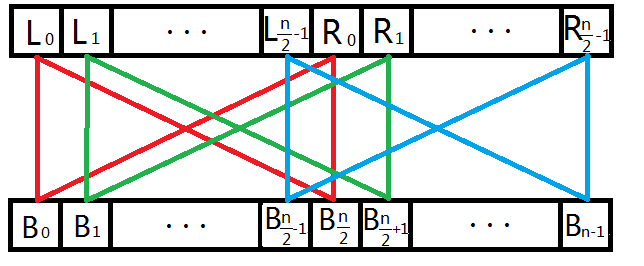我们根据上文关于信息合并的推导可知，L0${L}_{0}$$L_0$和R0${R}_{0}$$R_0$只决定B0${B}_{0}$$B_0$和Bn2